# Interval arithmetic facts for kids

Kids Encyclopedia Facts

Interval arithmetic is a specific type of computer arithmetic for (mathematical) intervals. It is mainly used for the automated detection of errors. There is a value, which isn't known exactly, but which can be given by an interval: the value is known to be inside the interval. There's a function to calculate the error. This function should be given the unknown value, but instead can only be given the interval. The result is a function which will map intervals.

Interval arithmetic can be used to treat rounding errors, or to treat insecurities with measurements: Each measurement has a certain error, which cannot be determined exactly.

## Definition

For real intervals (interval of real numbers), interval arithmetic is defined as follows:

• Addition: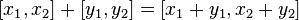$[x_1, x_2] + [y_1, y_2] = [x_1+y_1, x_2+y_2]$
• Subtraction: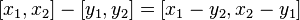$[x_1, x_2] - [y_1, y_2] = [x_1-y_2, x_2-y_1]$
• Multiplication: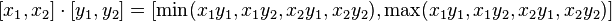$[x_1, x_2] \cdot [y_1, y_2] = [\min(x_1 y_1,x_1 y_2,x_2 y_1,x_2 y_2), \max(x_1 y_1,x_1 y_2,x_2 y_1,x_2 y_2)]$
• Division: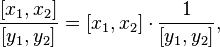$\frac{[x_1, x_2]}{[y_1, y_2]} = [x_1, x_2] \cdot \frac{1}{[y_1, y_2]},$
where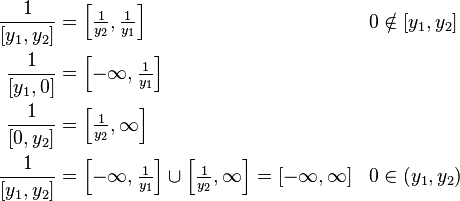\begin{align} \frac{1}{[y_1, y_2]} &= \left [\tfrac{1}{y_2}, \tfrac{1}{y_1} \right ] && 0 \notin [y_1, y_2] \\ \frac{1}{[y_1, 0]} &= \left [-\infty, \tfrac{1}{y_1} \right ] \\ \frac{1}{[0, y_2]} &= \left [\tfrac{1}{y_2}, \infty \right ] \\ \frac{1}{[y_1, y_2]} &= \left [-\infty, \tfrac{1}{y_1} \right ] \cup \left [\tfrac{1}{y_2}, \infty \right ] = [-\infty, \infty] && 0 \in (y_1, y_2) \end{align}

## Applications

Interval arithmetic is mainly used in the field of validated numerics. It is also used in other technical areas.

## Implementations